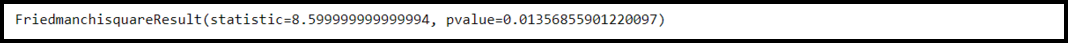Open in App
Not now

# How to Perform the Nemenyi Test in Python

• Last Updated : 02 Mar, 2022

Nemenyi Test: The Friedman Test is used to find whether there exists a significant difference between the means of more than two groups. In such groups, the same subjects show up in each group. If the p-value of the Friedman test turns out to be statistically significant then we can conduct the Nemenyi test to find exactly which groups are different. This test is also known as Nemenyi post-hoc test.

The Friedman Test follows the below hypothesis:

• The null hypothesis (H0): The mean value for each of the populations is equal.
• The alternative hypothesis: (Ha): At least one population mean differs from the others.

Syntax to install required libraries including scipy, scikit-posthocs, and NumPy:-

` pip3 install scipy scikit-posthocs numpy`

## Performing Nemenyi test in Python:

Step 1: Create the Data.

Let us consider an example in which researchers are interested to know if the mileage of cars is equal when doped with three different engine oils. In order to determine this, they measured the mileage (per kilometers) of 10 different cars on each of the three engine oils. We can create the following three arrays that contain the response times for each car when doped with each of the three engine oils.

## Python3

 `# Data groups ` `data_group1 ``=` `[``44``, ``56``, ``53``, ``46``, ``53``,` `               ``46``, ``42``, ``47``, ``46``, ``45``]` `data_group2 ``=` `[``35``, ``46``, ``38``, ``47``, ``37``,` `               ``38``, ``44``, ``46``, ``44``, ``35``]` `data_group3 ``=` `[``32``, ``42``, ``54``, ``43``, ``32``, ` `               ``32``, ``43``, ``36``, ``8``, ``29``]`

Step 2: Conduct the Friedman Test.

Now we will perform the Friedman test. scipy.stats library provides the friedmanchisquare() function to perform the Friedman test.

## Python3

 `# Importing library` `from` `scipy ``import` `stats`   `# Data groups` `data_group1 ``=` `[``44``, ``56``, ``53``, ``46``, ``53``, ` `               ``46``, ``42``, ``47``, ``46``, ``45``]` `data_group2 ``=` `[``35``, ``46``, ``38``, ``47``, ``37``,` `               ``38``, ``44``, ``46``, ``44``, ``35``]` `data_group3 ``=` `[``32``, ``42``, ``54``, ``43``, ``32``,` `               ``32``, ``43``, ``36``, ``8``, ``29``]`   `# Conduct the Friedman Test` `stats.friedmanchisquare(data_group1, data_group2, data_group3)`

Output:Friedman Test

Here, the test statistic comes out to be equal to 8.599 and the corresponding p-value comes out to be equal to 0.013. Since this p-value is less than 0.05, we can reject the null hypothesis that the mean mileage is the same for all three engine oils. In simple words, we have enough proof to say that the type of engine oil used produces statistically significant differences in response time.

Step 3: Conduct the Nemenyi Test.

Now, we can conduct the Nemenyi test to find exactly which groups have different means. scikit-posthocs library provides the posthoc_nemenyi_friedman() function using which we can conduct the Nemenyi test.

## Python3

 `# Importing libraries` `from` `scipy ``import` `stats` `import` `scikit_posthocs as sp` `import` `numpy as np`   `# Data groups` `data_group1 ``=` `[``44``, ``56``, ``53``, ``46``, ``53``,` `               ``46``, ``42``, ``47``, ``46``, ``45``]` `data_group2 ``=` `[``35``, ``46``, ``38``, ``47``, ``37``, ` `               ``38``, ``44``, ``46``, ``44``, ``35``]` `data_group3 ``=` `[``32``, ``42``, ``54``, ``43``, ``32``, ` `               ``32``, ``43``, ``36``, ``8``, ``29``]`   `# Conduct the Friedman Test` `stats.friedmanchisquare(data_group1, data_group2, data_group3)`   `# Combine three groups into one array` `data ``=` `np.array([data_group1, data_group2, data_group3])`   `# Conduct the Nemenyi post-hoc test` `sp.posthoc_nemenyi_friedman(data.T)`

Output:Nemenyi Test

We need to transpose the NumPy array (data.T) to conduct the post-hoc test efficiently. As you can see in the output, The Nemenyi post-hoc test produces the p-values for each pairwise comparison of means. These values are:

• P-value of group 0 vs. group 1: 0.503
• P-value of group 0 vs. group 2: 0.010
• P-value of group 1 vs. group 2: 0.173

For α = 0.05 there are only two groups that seem to have statistically significantly different means are group 0 and group 2.

My Personal Notes arrow_drop_up
Related Articles